# How to use the AND function in Excel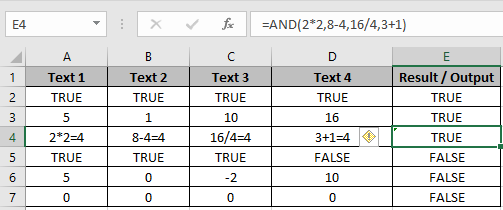In this article, we will learn How to use the AND function in Excel.

What is AND criteria ?

In Excel, we extract results based on the given criteria. For Example we need to extract the list of employees who are married and have salaries above half a million dollars per annum. The above criteria can be more difficult when there are more than 2 criterias. So Let's learn the AND function which checks each criteria and returns only and only if all stated criteria stands true. Returns False if any of the conditions is false. Use the AND function and the IF function along with AND function to get customized results for the required result.

AND Function in Excel

AND function returns TRUE or FALSE. Multiple criterias to match is obtained using the AND function. Mention the criterias inside the AND function separated using the ( , ).

Syntax:

 =AND(logical1, [logical2], [logical3], …)

logical1 : first criteria

logical2 : second criteria and further criteria goes on

Example :

All of these might be confusing to understand. Let's understand how to use the function using an example. Here we have few Examples to test multiple conditions and statements of AND function.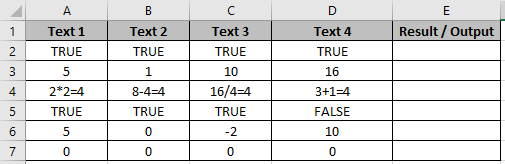Use the formula in E2 cell.

 =AND(A2,B2,C2,D2)

Press Enter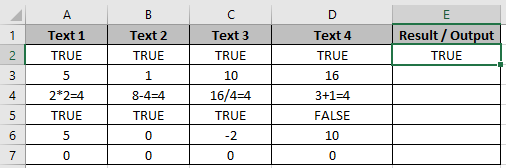It returns True as all the statements return True.

There are some default AND Excel statements

1. All numbers except 0 are considered True.
2. 0 is considered as False.
3. True is True and False is False.

Apply the formula in other cells using Ctrl + D taking the first applied cells till the cell you wish to copy formula.In the E2 cell, we applied the formula

 =AND(2*2,8-4,16/4,3+1)

We test the 4 conditions in this AND formula. All conditions return 4 which is considered True is Excel. If all conditions return positive numbers and any of it returns 0 then the formula will return False.

You can check less than or greater than condition on numbers. OR function can also be used along with many functions like IF, AND and many more.

IF - AND Function in Excel

IF function: You know how IF function in Excel works. It takes a boolean expression as the first argument and returns one expression IF TRUE and another if FALSE. Learn more about The Excel IF Function.

 =IF(TRUE or FALSE, statement if True, statement if false)

AND Function : Checks multiple conditions. Returns TRUE only if all conditions are TRUE else returns FALSE.

 =AND(condition1, condition2,....) ==> TRUE/FALSE

In the end, AND function provides IF function TRUE or FALSE argument and based on that IF prints the result.

Example

Here We have a list of Scores and We need to know under which criteria it lies.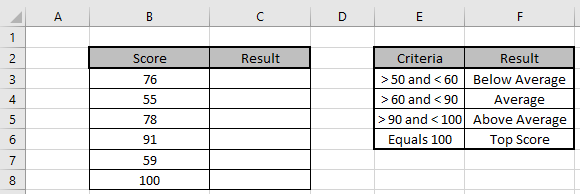Here multiple criterias are needed to satisfy. Use the formula to match the given criteria table

Formula:

 =IF(AND(B3 > 50, B3 < 60), "Below Average",IF(AND(B3 > 60, B3 < 90),"Average",IF(AND(B3 > 90,B3< 100),"Above Average","Top Score")))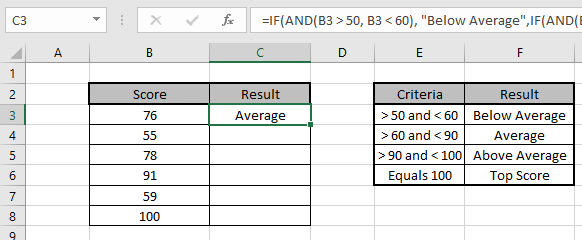Copy the formula in other cells, select the cells taking the first cell where the formula is already applied, use shortcut key Ctrl + D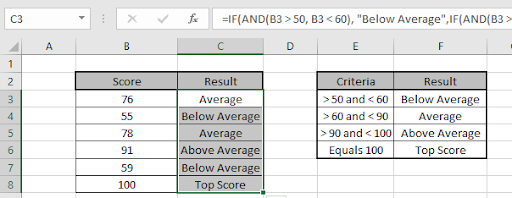We got the Results corresponding to the Scores.

You can use IF and AND function to meet multiple conditions in a single formula.

Here are all the observational notes using the AND function in Excel
Notes :

1. Make sure each condition in the formula works fine for the number values before using for all the other values.
2. Operations like equals to ( = ), less than equal to ( <= ), greater than ( > ) or not equals to ( <> ) can be performed within a formula applied, with numbers only.

Hope this article about How to use the AND function in Excel is explanatory. Find more articles on using logical values and related Excel formulas here. If you liked our blogs, share it with your friends on Facebook. And also you can follow us on Twitter and Facebook. We would love to hear from you, do let us know how we can improve, complement or innovate our work and make it better for you. Write to us at info@exceltip.com.

Related Articles :

How to Count Cells That Contain This Or That in Excel in Excel : To cells that contain this or that, we can use the SUMPRODUCT function. Here's how you do those calculations.

How to use nested IF function : nested IF function operates on data having multiple criteria. The use of repeated IF function is nested IF excel formula.

SUMIFS using AND-OR logic : Get the sum of numbers having multiple criteria applied using logic AND-OR excel function.

COUNTIFS With OR For Multiple Criteria : Count cells having multiple criteria match using the OR function. To put an OR logic in COUNTIFS function you will not need to use the OR function.

Using the IF with AND / OR Functions in Microsoft Excel : These logical functions are used to carry out multiple criteria calculations. With IF the OR and AND functions are used to include or exclude matches.

IF with OR function : Implementation of logic IF function with OR function to extract results having criteria in excel data.

IF with AND function : Implementation of logic IF function with AND function to extract results having criteria in Excel.

Popular Articles :

How to use the IF Function in Excel : The IF statement in Excel checks the condition and returns a specific value if the condition is TRUE or returns another specific value if FALSE.

How to use the VLOOKUP Function in Excel : This is one of the most used and popular functions of excel that is used to lookup value from different ranges and sheets.

How to use the SUMIF Function in Excel : This is another dashboard essential function. This helps you sum up values on specific conditions.

How to use the COUNTIF Function in Excel : Count values with conditions using this amazing function. You don't need to filter your data to count specific values. Countif function is essential to prepare your dashboard.

Terms and Conditions of use

The applications/code on this site are distributed as is and without warranties or liability. In no event shall the owner of the copyrights, or the authors of the applications/code be liable for any loss of profit, any problems or any damage resulting from the use or evaluation of the applications/code.